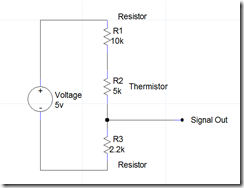How To Scale A Thermistor for An ArduinoA thermistor is a simple temperature component, basically it is a variable resistor that the resistance changes in it depending on the temperature. Such a simple device needs a few more components and scaling in the microcontroller to give the desired C or F temperature reading.

# Voltage dividerFirst you will need to build a voltage divider to convert the thermistors varying resistance to a varying voltage source to feed the analog input of the microcontroller. The voltage source and resister values can vary, all you want is to not pull too much current (use high value resistors) and provide a wide voltage range for the microcontroller for best accuracy.

# Scaling

The microcontroller will receive a raw value that will need to be scaled.

The zero and span of the raw value will vary depending on the voltage range of the analog input on your microcontroller. My Arduino analog input is 0-5vdc, some Arduinos are 0-3.3vdc, Industrial equipment is usually 1-5vdc 1-10VDC or 4-20ma.

The raw value inside the controller will vary depending on the resolution of the analog input, The Arduino Uno’s ADC (Analog to Digital Converter) is  is 10 bit so at 0vdc you will read 0 raw and at 5vdc you will read 1023 raw. Industrial ADCs often use 15 bit resolution so zero and span will be 0-32767. This raw number then must be scaled to what ever unit of measurement desired, in this case degrees Fahrenheit.

Scaling in the software usually requires Gain (multiplier) and Offset (add or subtract).

###### Gain

To figure out the gain we will use a proportion and cross multiply then divide the ratios. Example: We can run the temperature from 35-85 degrees using our hand for heat and ice in a bag, we check this with a thermometer. The raw values we see in the microcontroller are 417-565. We find the total range traveled by this equasion: 85-35=50 the range traveled of the raw value is: 565-417=148

Then lets use a proportion equation like this:  50/1 = 148/x to find x simply cross multiply and divide (1*148)/50=2.96. This is how much we will divide the raw number by.

Note: To be completely accurate with the definition of gain (multiplier) we would find the inverse so 1/2.96 = 0.33784 then multiply our raw number by it however I apologize, in my code example I just divided by 2.96, same result.  In the Arduino who cares but in some industrial applications they will expect an actual Gain(multiplier).

###### Offset

Offset is how much we need to move the value up or down. We will figure this on the lower values tested which was 35DegF-417raw. We will take into account that we will be dividing by 2.96 In this example equation: (417/2.96)-35=129.

# Fine tuning

Since the methods I used to running up and down the temperature were quite primitive and not very accurate (bag of ice and my hand verified with cheap thermometers) I expected to have some fine tuning to do. After you get the scale close you can tweak the Gain and Offset to get the reading as close as possible. Also read the data sheet on your thermistor, most are not exactly linier so even with the perfect Gain and Offset there will be error.

# Video

P.S. This scaling procedure works with just about any analog input, i.e temperature, pressure, voltage, humidity, etc..

# The Arduino Sketch

```int Ai0 = A0;
int Value0;

void setup()
{
Serial.begin(9600);
Serial.println("www.Electronhacks.com");
}

void loop()
{
Value0 = analogRead(Ai0); //Read the value of AI1 (pin0) and write it to Value0
Value0 = ((Value0/2.82)-108); //Scale the value

Serial.print("Raw-");